## Monday, 13 August 2012

### An open problem, matlab and randomness II

Before we deal with the original problem again, let's deal with the following problem:

Given X as a uniform distribution from 0 to 1. What's the expected value of E(1/X)?

Recall the formula of E(X) for uniform distribution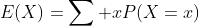When we extend the formula into continous distribution it becomes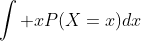.

Firstly we have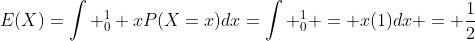.

Now how can we get the probability distribution for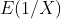?

Consider the following, let a and b be numbers within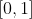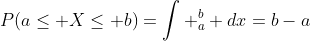Now take the inverse, let the inverse of X be Y: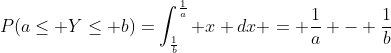. It's hard to deal with the integral form, but consider the probability density function with limits. Applying idea from calculus,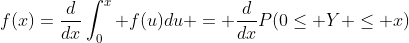which is FToC II (Fundamental Theorem of Calculus).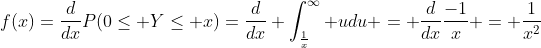Note that it isn't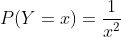because in a continuous probability density function the probability at a certain point must be zero.

Checking gives the desired answer: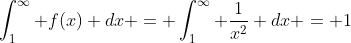so that it's a valid probability density function.

Now let's check the expected value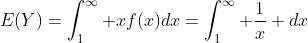which is equal to... errr... infinity?

However with simple matlab code you simply find that a finite value is returned:

------------
clear
total = 0
for I = 1:1e8
avg = (avg*(I-1)+rand)/I; %If you wish you can get the total before avg, but we try to avoid floating point turncating problem here.
end
avg
------------

With some trials usually we get values around 15~20 which is contradictory to our expectation. You don't believe you're making an error, so you plot the distribution of average of 1/X with some matlab code:

------------
clear
n=20000
avg=zeros(1,n);
count=zeros(1,100);
for J=1:n
for I=1:20000
y=rand;
y=1/y;
avg(J)=(avg(J)*(I-1)+y)/I;
end
end %loop taking average of 1/X
for J=1:n
for K=1:100
if avg(J)>=(K-1)&avg(J) < K
count(K) = count(K)+1
end
end
end %this is the counting machine
count = count/20000 %count -> probit
x=0.5:99.5
plot(x,count)
------------

Note that the above code takes quite a long time too compute. This is the graph plotted with the above code:

With such a clear result we don't really need to interpret the above result in detail, but what we noticed is that the expected value is different from what we get. Now think reversely. When we expect infinity when we find the reciprocal of number between 0 and 1? It's 0. Inversely if the number drawn is not close enough to zero the expected value is finite, and this should be true because all rand generated are non-zero and rational. Consider the following distribution:if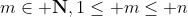which is the reciprocal of the dicrete version of m/n.

For this one we can calculate the expected value of X easily: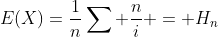where H is the partial harmonic sum. Assuming the inverse of uniform distrubtion is a limit of the above function:which tends to infinity, with the same speed with the above integral result!

(Note: this is not suprising.
1) The diverging speed is equal since the integral result gives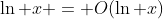and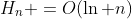as well. If you're not sure about the big O notation, check wikipedia or some passages in the past in this blog.

2) Of course they would converge in the same speed because they're only the discrete and continuous version of the same function. This is the core idea of the integral convergence test.)

With result of Euler we know that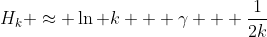, and then we can estimate the floating point limit of the computers.

In this section we discussed the basis of expected value with reciprocal operation, which will be useful in the coming sections. Last but not least, we'll bring out an very important inequality to avoid further mistakes: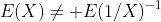This inequality works in most of the cases and is verified above.

Foods for thought:

1) Can you statistically describe the graph plotted above? Is it bionomial or poisson distribution, and why?
2) Use the above formula approximating Harmonic series to estimate the floating point limit of the computers.
3) If the floating point limit is extended (like from a single float to a double float), how do you expect the graph to shift? How about the case of infinity where the rand gives perfectly random numbers?